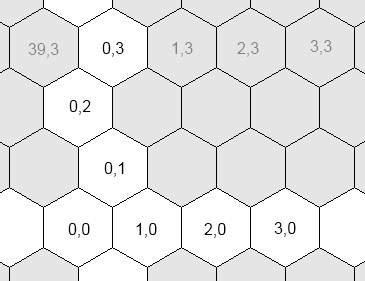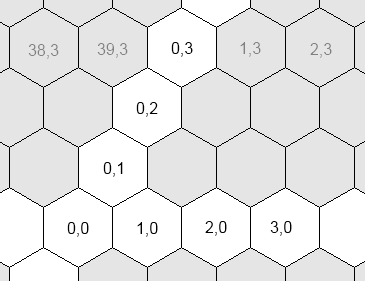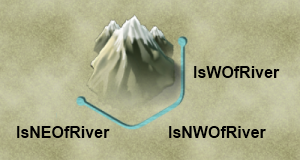# Map and terrain (Civ5)

Jump to: navigation, search

This page is a part of the Lua and UI Reference.

# Layout

### Coordinates systems

Civ5 uses three different coordinates systems for plots:

• The world coordinates. The coordinates of the plot's graphics in the 3D space where the world is rendered. This system is only used when you want to display an icon over the map, through a WorldAnchor.
• The grid coordinates. The logical coordinates of a plot. This is the system returned by Plot:GetX() and Plot:GetY()
• The hex coordinates. The logical coordinates of a plot. Some computations are easier to perform in this system.The grid coordinates: horizontal and vertical axes are orthogonal.The hex coordinates: the vertical axis makes a 60° angle with the horizontal axis.

The reason behind the coexistence of the grid and hex coordinates is because most computations are easier to perform in the hex system. For example, if you wish to examine the neighbors of a plot...

• In the grid coordinates, you first need to test whether y is odd or even. Depending on the result, the offsets of the top plots will be { (0,1), (1,1) } or { (-1,1), (0,1)}.
• However no such test is required in the hex system: the top offsets are always { (-1,1), (0,1) }.

### Helpful functions

The following global functions will help you:

 `Vector2` `Vector2(int x, int y)` makes a 2D vector. `int, int` `ToGridFromHex(int x, int y)` `int, int, int ` `GridToWorld(int x, int y)` `Vector2` `ToHexFromGrid(Vector2 hexPos)` `Vector3` ` HexToWorld(Vector2 hexPos)`

### Plots indexing

The function Map.GetPlotByIndex(int index) takes an index and returns a plot. This index can be computed as follow:

```local w = Map.GetGridSize()
local index = gridX + gridY * w```

### Enumerating neighbor plots

The best way is to use `Map.PlotDirection(int x, int y, DirectionType direction)`.

```local x, y = ... -- Initialize those
for i = 0, 5 do
local plot = Map.PlotDirection(x, y, i)
-- Do something with plot
end```

### Enumerating plots in a certain range

If you need to enumerate plots in specific orders, you should look at Border and plots iterator, a great library from Whoward69 who provides nice iterators for different scan orders.

Otherwise, Map.PlotXYWithRangeCheck offers a simple way to scan all plots within a certain range.

```local x, y = ... -- Initialize those
local range = 5
for dx = -range, range do
for dy = -range, range do
local plot = Map.PlotXYWithRangeCheck(x, y, dx, dy, range)
if plot then
-- Do whatever you want with this plot
end
end
end```

### The world is not flat

Maps can be wrapped along X or Y. You can get those values through Map.IsWrapX() and Map.IsWrapY().

• When wrapped along X, reaching the west side of the map brings you to the east side.
• When wrapped along Y, reaching the north side brings you to the south side.
• A map can neither be wrapped along X, neither around Y. For example a map that only covers a part of the world.
• A map cannot be both wrapped along X and Y.

# Terrain

### Nature

The nature, aspect and value of a Plot in civ5 are defined by the following data:

• PlotType. It can be ocean (includes lakes and coasts), land, hills or mountain.
• TerrainType. It can be ocean, coast (lake or coast), grasslands, plains, tundra or snow. A coast is automatically a lake if it belongs to a water area not larger than nine plots (see the next section).
• FeatureType. It can be none, forest, jungle, floodplains, marsh, oasis, ice, fallout or any natural wonder.
• ResourceType and a quantity value. It can be any resource: gold, gems, horses, fish, etc.
• ImprovementType and a "pillaged" boolean flag. It can be any improvement: mines, farm, etc. Goodies, city ruins and barbarian camps are also improvements.
• RouteType. It can be none, road or railroad.
• ArtStyleType. It can be South America, Asian, European, Middle-East, Polynesian Greco-Roman or Barbarian. It decides which graphics will be used to render the plot.
• Besides of those, a plot also stores its coordinates, references to the city and units it hosts, its owner index, and visibility flags for every player in the game.

### Areas

• The map is divided into different areas: one per landmass and one per watermass. That is, all plots in an island belong to the same area, which is different from the closest continent's area. Every lake or inner sea also has their own areas.
• Areas are represented in the API by the Area type.
• Water areas that contains nine plots at most are lakes. Otherwise they are oceans.
• Areas are computed when the map is generated, through Map.RecalculateAreas(). You should not recompute them unless you turn some plots from water to land and reciprocally.
• In Civ4 impassable plots had their distinct areas and they could diode a landmass into sub-areas. This is no longer true in civ5.

# Rivers

### MethodsYou can only query or modify three edges per plot: E, SE, SW

Every plot has:

• Three status getters:
• `bool Plot:IsWOfRiver()`. Checks the east edge (IsWOfRiver stands for "is at the west of a river")
• `bool Plot:IsNWOfRiver()`. SE edge.
• `bool Plot:IsNEOfRiver()`. SW edge.
• Three direction getters:
• `FlowDirectionType Plot:GetRiverEFlowDirection()`.
• `FlowDirectionType Plot:GetRiverSEFlowDirection()`.
• `FlowDirectionType Plot:GetRiverSWFlowDirection()`.
• Three setters:
• `Plot:SetWOfRiver(bool hasRiver, FlowDirectionType flow = -1)`.
• `Plot:SetNWOfRiver(bool hasRiver, FlowDirectionType flow = -1)`.
• `Plot:SetNEOfRiver(bool hasRiver, FlowDirectionType flow = -1)`.
• Two generalized getters that return true if there is a river in the specified direction (or towards the specified plot):
• `bool Plot:IsRiverCrossing(DirectionType direction)`
• `bool Plot:IsRiverCrossingToPlot(Plot toPlot)`

### Generalized functions

The fact that you can only query three edges is troublesome. Here are simple functions to circumvent this limitation. Note that the HasRiver function is redundant with the IsRiverCrossing and IsRiverCrossingToPlot methods.

```function HasRiver(plot, direction)
if direction == 1 then return plot:IsWOfRiver() end
if direction == 2 then return plot:IsNWOfRiver() end
if direction == 3 then return plot:IsNEOfRiver() end
local neighbor = Map.PlotDirection(plot:GetX(), plot:GetY(), direction)
return HasRiver(neighbor, direction - 3)
end

function GetRiverFlow(plot, direction)
if direction == 1 then return plot:GetRiverEFlowDirection() end
if direction == 2 then return plot:GetRiverSEFlowDirection() end
if direction == 3 then return plot:GetRiverSWFlowDirection() end
local neighbor = Map.PlotDirection(plot:GetX(), plot:GetY(), direction)
return GetRiverFlow(neighbor, direction - 3)
end

function SetRiver(plot, direction, hasRiver, flowDirection)
if direction == 1 then return plot:SetWOfRiver(hasRiver, flowDirection) end
if direction == 2 then return plot:SetNWOfRiver(hasRiver, flowDirection) end
if direction == 3 then return plot:SetNEOfRiver(hasRiver, flowDirection) end
local neighbor = Map.PlotDirection(plot:GetX(), plot:GetY(), direction)
return SetRiver(neighbor, direction - 3, hasRiver, flowDirection)
end```